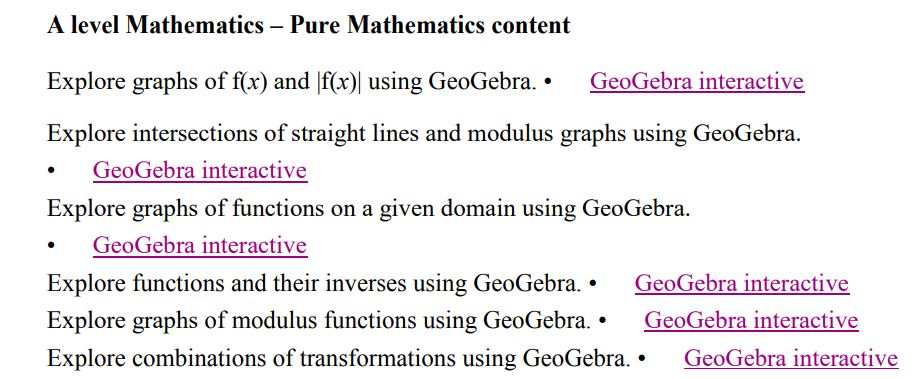# The Modulus Function

Looking at the Subject content for A Level Mathematics we see that students are expected to be able to understand and use graphs of functions including the modulus of a linear function.

Additionally, students should understand simple transformations of graphs.

This is an ideal opportunity to use graphing technology to understand and explore the modulus (or absolute value) function.

We can use Desmos to illustrate the functions f(x)=2x+3 and g(x)=|2x+3| illustrating that the left portion of g(x) is a reflection of the negative portion of f(x). We could also explore translations parallel to the x and y axes.

Looking at MEI’s excellent student tasks for A Level, we see that the a Modulus Function task is provided for Autograph, the Casio CG50 graphing calculator, Desmos and GeoGebra.

Like all the MEI student tasks instructions for the use of the software are provided some great questions to get students thinking and exploring.

Edexcel’s guide to using GeoGebra for AS and A Level Mathematics includes several GeoGebra Interactives for students to explore.Edexcel – Guide to using GeoGebra

This on exploring the intersection of lines and modulus graphs is very useful for solving equations involving modulus functions.Edexcel – guide to GeoGebra for A Level Mathematics

There are several sources of notes and examples as well as worked exam questions on this topic. Explore these below – a reminder of the many excellent sites available.

Of course we have to start with the outstanding Dr Frost Maths, (you might have seen him in the news recently!) where you will find resources on the Modulus Function under Graphs and Functions for Key Stage 5. Scroll down for “Sketch the modulus of a linear function.”

Owen (Owen134866 on TES) has a library of Mathematics Further Mathematics teaching resources, these are really clearly structured with step by step examples. Functions and Graphs includes the modulus function and can be found on TES in his A Level Pure Mathematics Year 2 Collection.Exam Solutions

On Exam Solutions we have four worked examination questions on Modulus Inequalities, additionally there are two helpful tutorials, one is an introduction to the modulus function and the other on Graphing y=|f(x)|.HELM Notes – Some Common Functions

One of the series of excellent HELM Notes is Some Common Functions under Section 2 on Basic Functions; the modulus function is briefly covered in Section 3 of the document, a worked example is provided to plot a modulus function.Maths Genie – Functions

On Maths Genie the questions and solutions on Functions includes one question (7) on the Modulus function. Students should be able to solve problems such as finding the values of x which satisfy │2x – 3│< 9; they should be able to do this both algebraically and graphically.

From the Math Centre, we have a first aid kit on the modulus symbol.